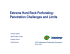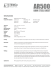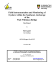# MINISTRY OF SCIENCE AND TECHNOLOGY DEPARTMENT OF TECHNICAL AND VOCATIONAL EDUCATION

## Transcription

MINISTRY OF SCIENCE AND TECHNOLOGY DEPARTMENT OF TECHNICAL AND VOCATIONAL EDUCATION
```MINISTRY OF SCIENCE AND TECHNOLOGY
DEPARTMENT OF
TECHNICAL AND VOCATIONAL EDUCATION
SAMPLE QUESTIONS &
WORKED-OUT EXAMPLES FOR
CE – 03014
DESIGN OF TIMBER STRUCTURES
B.Tech. (First Year)
Civil Engineering
MINISTRY OF SCIENCE AND TECHNOLOGY
DEPARTMENT OF
TECHNICAL AND VOCATIONAL EDUCATION
CE-03014
DESIGN OF TIMBER STRUCTURES
SAMPLE QUESTIONS
B.Tech. (First Year)
Civil Engineering
Note
*
Must Know
**
Should Know
*** Could Know
1
Yangon Technological University
Department of Civil Engineering
Course No. CE 05024 + CE 06024 Design of Timber Structures I + II
Sample Questions
CE 05024 Design of Timber Structures I
Chapter 1
1.3 Design of Compression Member
1.*
Determine an acceptable section surfaced- four-sides (S4S), for a laterallybraced double-hinged column 14 ft long, subjected to an axial load P = 40
Kips due to snow-loading conditions ( α = 1.15). The column is to be used in
an exposed structure and may be subjected to wetting for an extended period
of time. Use wood pressure- impregnated with preservatives. Consider wood
for which Fc = 1.3 ksi, Fb = 1.6 ksi and E = 1800 ksi. (Ans: 8 x 8 S4S timber)
2.*
Determine an acceptable section, surfaced- four-sides (S4S), for a column 14 ft
long subjected to the combined action of longitudinal load P = 40 Kips with an
eccentricity of 3 in at each end and a uniformly distributed transverse load of
0.245 Kips per ft, all due to normal loading conditions ( α = 1.00). Sidesway
of the column is prevented by bracing at the end supports but rotation of the
latter is unrestrained. The column is to be used in a covered structure and will
remain dry thereby requiring no downward adjustments in the allowable
stresses to increasing moisture content. Use wood for which Fc = 1.3 ksi,
Fb = 1.6 ksi and E = 1800 ksi. (Ans: 8 x 14 S4S timber)
2
3.*
Determine an acceptable section for a double- hinged braced column subjected
to P = 40 K, e = 3 in, w = 0.245 K/ft, and made out of wood for which Fc = 1.3
Ksi, Fb = 1.6 ksi and E = 1800 ksi. Consider that wind- loading conditions
apply (α = 1.33) and the column is braced at midheight by wood girts
attached to the wide face of the section. (Ans: 6 x 14 S4S section)
4.*
Determine three acceptable S4S sections, for a column 19 ft long subjected to
the combined action of longitudinal load P = 40 Kips with an eccentricity of
3 in., and a midspan moment M = 72 K-in due to transverse loading. Assume
α = 1.0 and such wood that Fc = 1.3 ksi, Fb = 1.6 ksi and E = 1800 ksi. The
column is to be used in a covered structure, hence, no adjustments in the
allowable stresses are needed.
(Ans: 10 x 14, α = 0.825; 8 x 18, α = 0.978; 12 x 12, α = 0.859)
5.*
Determine three acceptable S4S sections, for a column 10 ft long subjected to
the combined action of longitudinal load P = 40 Kips with an eccentricity of
3 in., and a midspan moment M = 72 K-in due to transverse loading. Assume
3
α = 1.0 and such wood that Fc = 1.3 ksi, Fb = 1.6 ksi and E = 1800 ksi. The
column is to be used in a covered structure, hence, no adjustments in the
allowable stresses are needed.
(Ans: 6 x 18, α = 0.843; 8 x 14, α = 0.856; 10 x 12, α = 0.861)
6.**
Design a spaced column as a braced double-hinged member for which the
effective length is the actual length. Consider using glued- laminated wood for
which Fc = 2.2 ksi, Fb = 2.2 ksi for load parallel to wide face of laminations, Fb
= 2.6 ksi for load perpendicular to wide face of laminations and E = 2000 ksi,
P = 104 Kips and length = 24.8 ft. Assume snow-loading condition.
(Ans: Two 6.75 x 12 in)
7.**
Design a solid column as a braced double-hinged member for which the
effective length is the actual length. Consider using glued- laminated wood for
which Fc = 2.2 ksi, Fb = 2.2 ksi for load parallel to wide face of laminations, Fb
= 2.6 ksi for load perpendicular to wide face of laminations and E = 2000 ksi,
P = 104 Kips and length = 24.8 ft. Assume snow-loading condition.
8.*** The interior column of a building supports the box-girder at its continuous
end. The column is subjected to a load equal to P = 41.22 Kips, which is
transmitted to it by direct compression between column and box-beam. The
length of the column is 21 ft from finished floor elevation to bottom of boxgirder.
Column
may
be
considered
braced
against
relative
lateral
displacements of the ends and a solid timber section, S4S wood having
allowable stresses as follows: Fb = 1.65 ksi, Fc = 1.05 ksi, and E = 1600 ksi.
Assuming that it is hinged at both ends about both axes and that it is subjected
to end moments created by a specified minimum eccentricity of the load.
(Ans: 10 x 10 S4S section, α = 0.92)
9.*** The interior column of a building is subjected to a load equal to P = 41.22
Kips. The length of the column is 21 ft from finished floor elevation to bottom
of box- girder. Design the column assuming that it is hinged to the foundation
4
pedestal, but fully fixed to the supported beam. Consider as a solid timber
section, S4S wood having allowable stresses as follows: Fb = 1.65 ksi,
Fc = 1.05 ksi and E = 1600 ksi. Consider two cases: (a) full lateral bracing, and
10.*** The interior column of a building is subjected to a load equal to P = 41.22
Kips. The length of the column is 21 ft from finished floor elevation to bottom
of box-girder. Design the column assuming that it is rotationally fixed to both
the foundation pedestal and the supported girder. Design for (a) full lateral
bracing and (b) no lateral bracing. Consider as a solid timber section, S4S
wood having allowable stresses as follows: Fb = 1.65 ksi, Fc = 1.05 ksi and E
5
1.4 Design of Bending Members
1.*
Check the capacity in bending of a system of 4 x 10 surfaced-four-sides floor
beams spaced 2 ft apart. Each beam supports 50 plf of uniformly distributed
load which includes its own weight, plus the weight of a 4 in wood deck nailed
to it. In addition, any beam is subject to a moving concentrated load of 6 Kips
due to normal loading conditions. The span is 10 ft and the wood is such that
allowable stresses in bending are Fb = 1.95 ksi engineered uses and 2.25 ksi
repetitive member use. Wood is used at 15% maximum moisture constant.
(Ans: The beam meets the requirements for flexure).
2.*
Check the capacity against horizontal shear stress of the beam of a system of 4
x 10 S4S floor beams spaced 2ft apart. Each beam supports 50 plf of
uniformly distributed load which includes its own weight, plus the weight of a
4 in wood deck nailed to it. In addition, any beam is subjected to a moving
ft and the wood is such that allowable stress in shear is 0.095 ksi. (Ans: The
beam meets the requirements for shear).
3.*
Determine the maximum deflection of the beam of a system of 4 x 10 S4S
floor beams spaced 2 ft. apart. Each beam supports 50 plf of uniformly
distributed load which includes its own weight, plus the weight of a 4 inch
wood deck nailed to it. In addition, any beam is subject to a moving
ft and the modulus of elasticity of the wood is 1900 ksi. (Ans: Total deflection,
δtot : = 0.3 in)
4.*
A system of 4 x 10 S4S floor beams spaced 2 ft apart and each beam supports
50 plf of uniformly distributed load which includes its own weight, plus the
weight of a 4 inch wood deck nailed to it. In addition, any beam is subject to a
span is 10 ft and the beams are supported in end bearing by a 6 x 24 in glued
6
laminated girder, to which they are toe-nailed. Determine the suitability of the
support. (Ans: The beam has adequate support.)
5.*
Design a double-tapered straight glued- laminated roof beam to meet the
following requirements: span 60 ft, spacing 16 ft, roof slope 1/12, dead load
(including self-weight of the beam) 15 psf, and snow load 30 psf; maximum
deflection limited to 1/180th of the span, i.e. 4 in. The roof decking is applied
directly to the beams, thus providing continuous lateral support to the top,
compression flange. Assume the following allowable stresses: Fb = 2.4 ksi,
Fv = 0.165 ksi, Fc⊥ = 0.415 ksi, E = 1800 ksi, G = E/16 = 112.5 ksi. Provide a
minimum camber equal to 1 and ½ times the dead load deflection. (Ans: b =
51 /8 '', do = 30'', dc = 60'')
6.*
A floor is supported by 2 x 12 in joists, at 16 in spacing, on a 16 ft span. The
joists are of a wood species and moisture content such that allowable stresses
are: Fb = 1.90 ksi, for repetitive- member uses; Ft = 1.10 ksi; Fv = 0.095 ksi; Fc⊥
= 0.405 ksi; Fc = 1.45 ksi; and E = 1900 ksi. Assume normal loading
conditions, continuous lateral support and full bearing on 6 in. (nominal) wide
girders. The maximum deflection under the total load should not exceed
1/240th of the span or 1 in. Determine the allowable uniform load, including
self-weight, that the joists may take and specify whether it depends on
bending, shear, end bearing or deflection.
7.*
A glued- laminated beam 6.75 in wide x 30 in deep spans 40 ft. simply
supported. It carries the following uniformly distributed load. wDL = 0.1 K/ft.,
which includes its own weight, and wLL = 0.7 K/ft.; the beam is braced
laterally at 5 ft. spacing. Assume normal loading conditions and dry conditions
of use. Allowable stresses are: F b = 2.4 ksi; Ft = 1.6 ksi; Fc = 1.5 ksi;
Fc⊥ = 0.45 ksi and 0.385; for tension face and compression face, respectively;
Fv = 0.165 ksi and E = 1800 ksi. Determine the following items:
(i)
Section properties.
(ii) Design moment and shear, reaction at support.
(iv) Check lateral stability.
7
(v) Allowable moment and shear.
Ans: (i) A = 202 in2 ., S = 1012 in3 ., I = 15187 in4 ., CF = 0.903.
(ii) M = 1920 K-in, V = 14 K, R = 16 K.
(iii) δ DL = 0.21 in., δT L= 1.69 in., 3/8 in. camber at midspan.
(iv) le = 115 in., CS = 8.7, CK = 21.2, C3 < 10, Fb = 2.4 ksi.
(v) M = 2190 K-in., V = 22.3 K.
8.**
Design a double-tapered straight glued- laminated roof beam to meet the
following requirements: span 60 ft, spacing 12 ft apart, roof slope 1/12, dead
load (including self- weight of the beam) 15 psf, and snow load 30 psf;
maximum deflection limited to 1/180th of the span, i.e. 4 in. The 3 in thick roof
decking is applied directly to the beams, thus providing cont inuous lateral
support to the top, compression flange. Assume the following allowable
stresses: Fb = 2.4 ksi, Fv = 0.165 ksi, Fc⊥= 0.415 ksi, E = 1800 ksi, G = E/16 =
112.5 ksi. Provide a minimum comber equal to 1 and ½ times the dead load
deflection.
9.**
Twenty feet long solid-sawn timber beams, spaced 8 ft-apart and simply
supported on masonry walls ( 6 in at each end ), carry a roof deck nailed
directly to the beams. The loads are uniformly distributed and consist of the
dead load of the deck and roofing materials, estimated at 10 psf, and the
design snow load of 30 psf. Consider the beams made of wood having the
following allowable stresses: Fb = 1.50 ksi, Ft = 1.00 ksi, Fv = 0.09 ksi,
Fc⊥= 0.40 ksi, Fc = 1.25 ksi, and E = 1800 ksi. Determine the adequacy of the
design, if the beams are 4 x 16's.
10.*** Design a simply-supported, glued- laminated roof beam to meet the following
requirements: span, L = 50 ft; spacing, s = 20 ft; dead load of supported joists
(on 20 in centers) and nailed sheathing WDL = 10 psf; snow load on roof
WSL = 30 psf; deflection limits, δTL = L/180; camber, c = 1.5 δ DL. Use wood
with the following allowable stresses: Fb = 2.6 ksi, Fv = 0.165 ksi, Fc⊥ = 0.45
ksi and E = 1800 ksi. Consider the top flange of the beam braced laterally by
the joists.
8
Chapter 2
Mechanical Fasteners and Connectors
1.*
Determine the allowable loads in compression parallel and perpendicular to
grain and at a 45° angle of load to grain, of a ¾ in. bolt in a 3 in. thick timber
having basic stresses of 1450 psi and 320 psi compression parallel and
perpendicular to grain, respectively. (Ans: P = 3000 lb, 1010 lb and 1500 lb.)
2.*
A 3 x 6 in S4S Douglas fir member fabricated at 15% moisture content is
spliced with two 2 x 6 side plates by means of six ¾ in. bolts, as shown in Fig.
The member is part of a structure that is used under cover and for which the
moisture content will remain at 15%. The force T in the member is caused by
snow- loading conditions. Determine the maximum value of T that the splice
may take. (Ans: T = 13400 lb.)
3.*
A 3 x 6 in. S4S Douglas fir member fabricated at 15% moisture content is
spliced with two A 36 steel plates instead of wood side members, as shown in
Fig. The member is part of a structure that is used under cover and for which
the moisture content will remain at 15%. The force T = 13.4 Kips in the
member is caused by snow- loading conditions. Design the connection using ¾
in. bolts.
9
(a) Semi- Elevation of splice
4.*
(b) Section A-A
A 4 x 4 in. tie is fastened to a 4 x 8 in. beam by two ¼ in. steel side plates and
¾ in. bolts as shown in Fig. Wood is surfaced-four-sides Southern pine No. 1,
surfaced dry and used at 19% maximum moisture content. The connection
suspended vertical tie to the beam. Determine the number of bolts required
and check the adequacy of the connection. (Ans: Use 2 bolts in tie and 4 bolts
in the beam.)
5.*
A 4 x 4 in. tie is fastened to a 4 x 8 in. beam by two ¼ in. steel side plates and
¼ in. bolts as shown in Fig. Wood is surfaced-four-sides Southern pine No. 1,
surfaced dry and used at 19% maximum moisture content. The connection
```

### From the Inside, Out! ENG.indd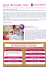### timetal 35a - PCC Energy Group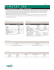### Sample Calculations – Connection 2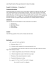### LUCKY YOU. - MonsieurMuffler.com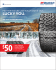### Extreme Hard Rock Perforating: Penetration Challenges and Limits